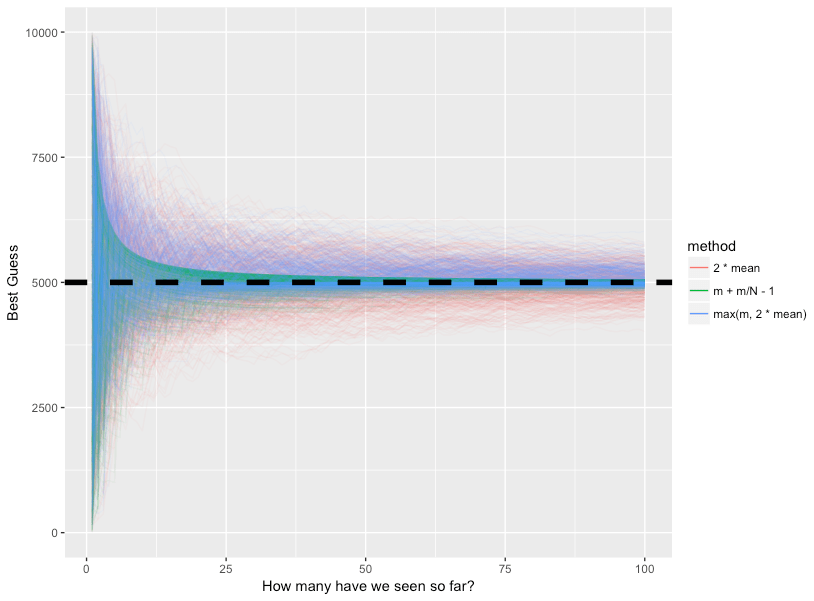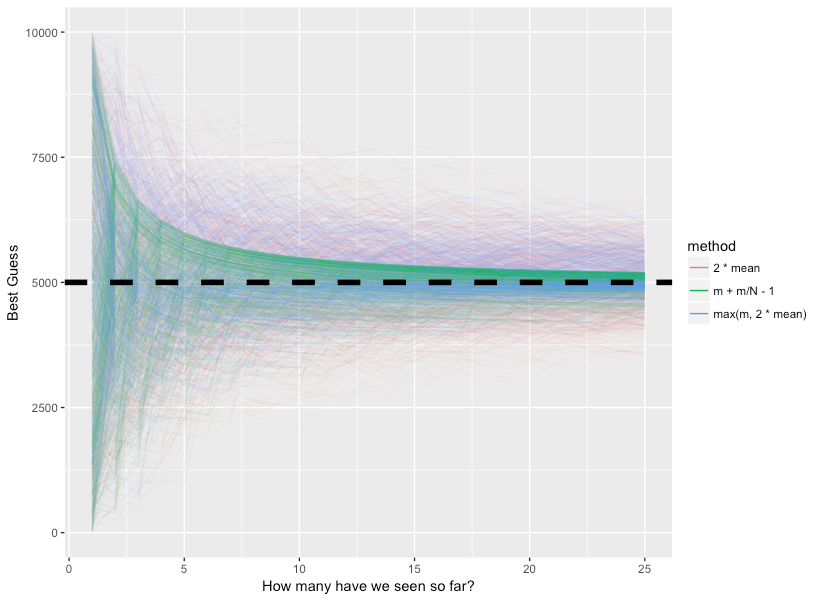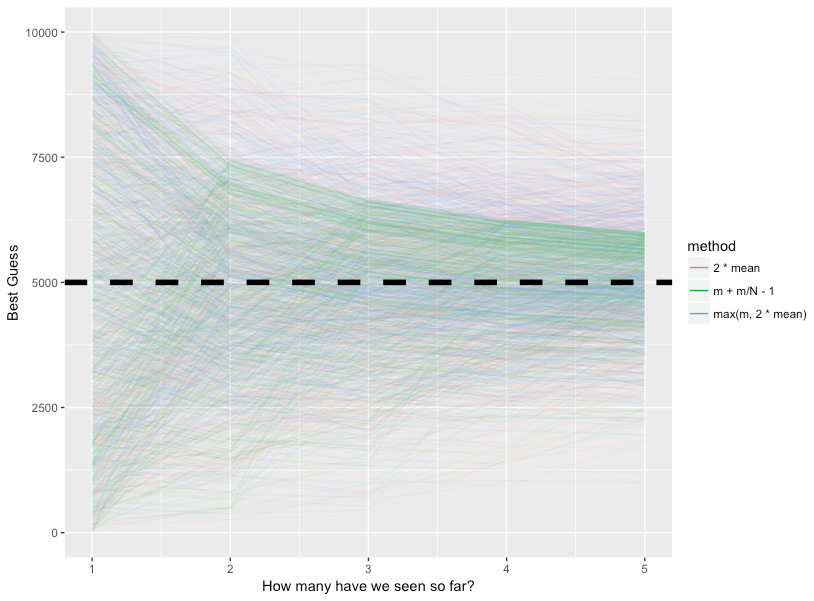Want to share your content on R-bloggers? click here if you have a blog, or here if you don't.

I always get giddy when I can apply real statistics and math to problems in my life. Recently, I had an opportunity to apply the ‘Taxicab Problem’ to something that came up at work. Given that I work for a ridesharing platform and I was quite literally counting “taxis” (or at least cars meant to drive others around), this was doubly exquisite.

For the uninitiated, the Taxicab / Germany Tank problem is as follows:

Viewing a city from the train, you see a taxi numbered x. Assuming taxicabs are consecutively numbered, how many taxicabs are in the city?

This was also applied to counting German tanks in World War II to know when/if to attack. Statstical methods ended up being accurate within a few tanks (on a scale of 200-300) while “intelligence” (unintelligence) operations overestimated numbers about 6-7x. Read the full details on Wikipedia here (and donate while you’re over there).

Somebody suggested using $2 * \bar{x}$ where $\bar{x}$ is the mean of the current observations. This made me feel all sorts of weird inside but I couldn’t quite put my finger on what was immediately wrong with this (outside of the glaringly obvious, touched upon shortly). On one hand, it makes some sense intuitively. If the distribution of the numbers you see is random, you can be somewhat sure that the mean of your subset is approxiately the mean of the true distribution. Then you simply multiply by 2 to get the max since the distribution is uniform. However, this doesn’t take into account that $2 * \bar{x}$ might be lower than $m$ where $m$ is the max number you’ve seen so far. An easy fix to this method is to simply use $max(m, 2 * \bar{x})$.

To be most correct, we can use the MVUE (Minimum Variance Unbiased Estimator). I won’t bore you with the derivation (and it’s also on the Wiki), but it works out to be $m + \frac{m}{N} – 1$ with $m$ is again the max number you’ve seen and $N$ is the total number you’ve seen. Let’s compare all 3 methods below:

Let’s plot it:Zoom in a bit:And this one is just prettttyyyy:As we can see, the green line converges to the true max of 5000 very quickly, and has far less variance than the two other methods. The modified method I proposed is just an asymmetric version of $2 * \bar{x}$, which approaches the true max but seems to level out in local minima/maxima at certain points. Ultimately, you can’t deny that the MVUE method is best.

Another approach we can take is a Bayesian one. Since I’d never pass up an opportunity to showcase my R package, bayesAB, (github repo here) for Bayesian AB testing let’s work through an example with bayesAB. This is actually an extra cool example since it showcases that while bayesAB is primarily meant to fit and compare two sets of posteriors, it can still be used to fit a single Bayesian model.

For brevity’s sake, we fit a Bayesian model using the Pareto distribution as the conjugate prior (if this is all a foreign language to you, definitely check out my other blog posts on bayesAB 1 2). We can use very small values for xm and alpha as diffuse priors so the model we build for the Uniform distribution is solely based on the data.As we can see, the posterior is fairly thin between 4980 and 5000. Taking the mean or median of this is a solid estimate for the maximum. Let’s see what the median says using the summary generic.

For our purposes, we can ignore everything besides the quantiles of the posteriors. Using this, we arrive at an estimate of 4983 as our max. Using $2 * \bar{x}$ for this same sample results in 5034 which is overshooting by quite a bit.